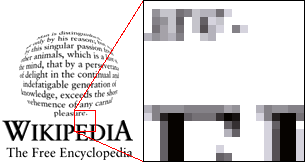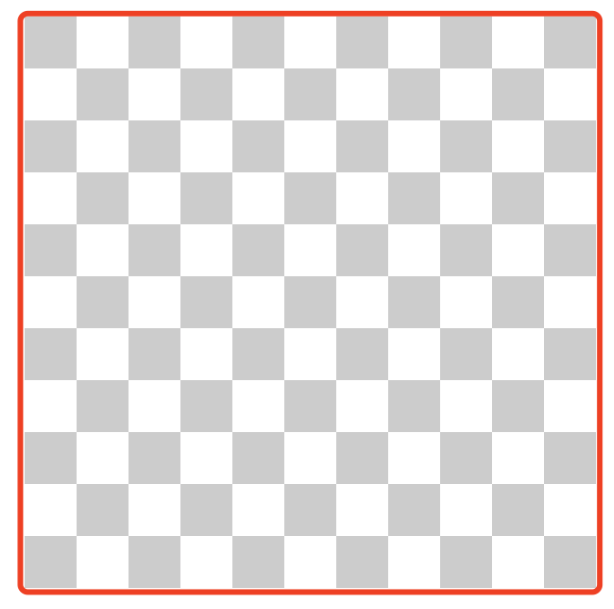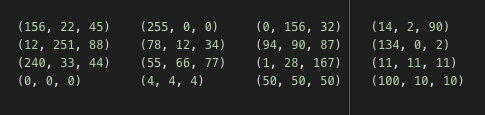#.

Yupoo Picture Manager serves tens of millions of users and manages tens of billions of pictures. As its user gallery is growing larger, Yupoo has an urgent business need for a solution that can quickly locate the image. In other words, when a user inputs an image, the system should find its original image and similar images in the gallery. The development of the search by image service provides an effective approach to this problem.

The search by image service has undergone two evolutions:

1. Began the first technical investigation in early 2019 and launched the first-generation system in March and April 2019;
2. Began the investigation of the upgrade plan in early 2020 and started the overall upgrade to the second-generation system in April 2020.

This article describes the technology selection and basic principles behind the two generations of search by image system based on my own experience on this project.

# Overview

What is an image?

We must know what is an image before dealing with images.

The answer is that an image is a collection of pixels.

For example, the part in the red box on this image is virtually a series of pixels.Suppose the part in the red box is an image, then each independent small square in the image is a pixel, the basic information unit. Then, the size of the image is 11 x 11 px.## Mathematical representation of images

Each image can be represented by a matrix. Each pixel in the image corresponds to an element in the matrix.

## Binary images

The pixels of a binary image is either black or white, so each pixel can be represented by 0 or 1.

For example, the matrix representation of a 4 * 4 binary image is:

```0 1 0 11 0 0 01 1 1 0
0 0 1 0```

RGB images

The three primary colors (red, green, and blue) can be mixed to produce any color. For RGB images, each pixel has the basic information of three RGB channels. Similarly, if each channel uses an 8-bit number (in 256 levels) to represent its gray scale, then the mathematical representation of a pixel is:

`([0 .. 255], [0 .. 255], [0 .. 255])`

Taking a 4 * 4 RGB image as an example:The essence of image processing is to process these pixel matrices.

# The technical problem of search by image

If you are looking for the original image, that is, an image with exactly the same pixels, then you can directly compare their MD5 values. However, images uploaded to the Internet are often compressed or watermarked. Even a small change in an image can create a different MD5 result. As long as there is inconsistency in pixels, it is impossible to find the original image.

For a search-by-image system, we want to search for images with similar content. Then, we need to solve two basic problems:

• Represent or abstract an image as a data format that can be processed by a computer.
• The data must be comparable for calculation.

More specifically, we need the following features:

• Image feature extraction.
• Feature calculation (similarity calculation).

# The first-generation search-by-image system

Feature extraction — image abstraction

The first-generation search-by-image system uses Perceptual hash or pHash algorithm for feature extraction. What are the basics of this algorithm?

As shown in the figure above, the pHash algorithm performs a series of transformations on the image to get the hash value. During the transformation process, the algorithm continuously abstract images, thereby pushing the results of similar images closer to each other.

## Feature calculation — similarity calculation

How to calculate the similarity between the pHash values of two images? The answer is to use the Hamming distance. The smaller the Hamming distance, the more similar the images’ content.

What is Hamming distance? It is the number of different bits.

For example,

`Value 1： 0 1 0 1 0Value 2： 0 0 0 1 1`

There are two different bits in the above two values, so the Hamming distance between them is 2.

Now we know the principle of similarity calculation. The next question is, how to calculate the Hamming distances of 100-million-scale data from 100-million-scale pictures? In short, how to search for similar images?

In the early stage of the project, I did not find a satisfactory tool (or a computing engine) that can quickly calculate the Hamming distance. So I changed my plan.

My idea is that if the Hamming distance of two pHash values is small, then I can cut the pHash values and the corresponding small parts are likely to be equal.

For example,

`Value 1： 8 a 0 3 0 3 f 6Value 2： 8 a 0 3 0 3 d 8`

We divide the above two values into eight segments and the values of six segments are exactly the same. It can be inferred that their Hamming distance is close and thus these two images are similar.

After the transformation, you can find that the problem of calculating Hamming distance has become a problem of matching equivalence. If I divide each pHash value into eight segments, as long as there are more than five segments that have exactly the same values, then the two pHash values are similar.

Thus it is very simple to solve equivalence matching. We can use the classical filtering of a traditional database system.

Of course, I use the multi-term matching and specify the degree of matching using minimum_should_match in ElasticSearch (this article does not introduce the principle of ES, you can learn it by yourself).

Why do we choose ElasticSearch? First, it provides the above-mentioned search function. Second, the image manager project in itself is using ES to provide a full-text search function and it is very economical to use the existing resources.

# Summary of the first-generation system

The first-generation search-by-image system chooses the `pHash + ElasticSearch` solution, which has the following features:

• The pHash algorithm is simple to use and can resist a certain degree of compression, watermark, and noise.
• ElasticSearch uses the existing resources of the project without adding additional costs to the search.

But the limitation of this system is obvious: The pHash algorithm is an abstract representation of the entire image. Once we destroy the integrity of the image, such as adding a black border to the original image, it is almost impossible to judge the similarity between the original and the others.

To break through such limitations, the second-generation image search system with a completely different underlying technology emerged.

## The Second-Generation Search-by-Image System

The second-generation search-by-image system technically chooses the `CNN + Milvus` solution. The system is based on feature vectors and provides better technical support.

### Feature Extraction

In the field of computer vision, the use of artificial intelligence has become the mainstream. Similarly, the feature extraction of the second-generation search-by-image system uses convolutional neural network (CNN) as the underlying technology.

The term CNN is difficult to understand. Here we focus on answering two questions:

• What can CNN do?
• Why can I use CNN for an image search?

There are many competitions in the AI field and image classification is one of the most important. The job of image classification is to determine whether the content of the picture is about a cat, a dog, an apple, a pear, or other types of objects.

What can CNN do? It can extract features and recognize objects. It extracts features from multiple dimensions and measures how close the features of an image are to the features of cats or dogs. We can choose the closest ones as our identification result which indicates whether the content of a specific image is about a cat, a dog, or something else.

What is the connection between the object identification function of CNN and search by image? What we want is not the final identification result, but the feature vector extracted from multiple dimensions. The feature vectors of two images with similar content must be close.

## Which CNN Model should I Use?

The answer is VGG16. Why choose it? First, VGG16 has good generalization capability, that is, it is very versatile. Second, the feature vectors extracted by VGG16 have 512 dimensions. If there are very few dimensions, the accuracy may be affected. If there are too many dimensions, the cost of storing and calculating these feature vectors is relatively high.

Using CNN to extract image features is a mainstream solution. We can use VGG16 as the model and Keras + TensorFlow for technical implementation. Here is the official example of Keras:

``

The features extracted here are feature vectors.

### 1. Normalization

To facilitate subsequent operations, we often normalize feature:

What is used subsequently is also the normalized `norm_feat`.

### 2. Image Description

The image is loaded using the`image.load_img` method of `keras.preprocessing`

In fact, it is the TensorFlow method called by Keras. For details, see the TensorFlow documentation. The final image object is actually a PIL Image instance (the PIL used by TensorFlow).

### 3. Bytes Conversion

In practical terms, image content is often transmitted through the network. Therefore, instead of loading images from path, we prefer converting bytes data directly into image objects, that is, PIL Images:

The above `img` is the same as the result obtained by the `image.load_img` method. There are two things to pay attention to:

• You must do RGB conversion.

• You must resize (resize is the second parameter of the `load_img` method).

### 4. Black Border Processing

Images, such as screenshots, may occasionally have quite a few black borders. These black borders have no practical value and cause much interference. For this reason, removing black borders is also a common practice.

A black border is essentially a row or column of pixels where all pixels are (0, 0, 0) (RGB image). To remove the black border is to find these rows or columns and delete them. This is actually a 3-D matrix multiplication in NumPy.

An example of removing horizontal black borders:

This is pretty much what I want to talk about using CNN to extract image features and implement other image processing. Now let’s take a look at vector search engines.

### Vector Search Engine

The problem of extracting feature vectors from images has been solved. Then the remaining problems are:

• How to store feature vectors?
• How to calculate the similarity of feature vectors, that is, how to search?

The open-source vector search engine Milvus can solve these two problems. So far, it has been running well in our production environment.

## Milvus, the Vector Search Engine

Extracting feature vectors from an image is far from enough. We also need to dynamically manage these feature vectors (addition, deletion, and update), calculate the similarity of the vectors, and return the vector data in the nearest neighbor range. The open-source vector search engine Milvus performs these tasks quite well.

The rest of this article will describe specific practices and points to be noted.

### 1. Requirements for CPU

To use Milvus, your CPU must support the avx2 instruction set. For Linux systems, use the following command to check which instruction sets your CPU supports:

`cat /proc/cpuinfo | grep flags`

Then you get something like:

What follows flags is the instruction sets your CPU supports. Of course, these are much more than I need. I just want to see if a specific instruction set, such as avx2, is supported. Just add a grep to filter it:

`cat /proc/cpuinfo | grep flags | grep avx2 `

If no result is returned, it means that this specific instruction set is not supported. You need to change your machine then.

### 2. Capacity Planning

Capacity planning is our first consideration when we design a system. How much data do we need to store? How much memory and disk space does the data require?

Let’s do some quick maths. Each dimension of a vector is float32. A float32 type takes up 4 Bytes. Then a vector of 512 dimensions requires 2 KB of storage.  By the same token:

• One thousand 512-dimensional vectors require 2 MB of storage.
• One million 512-dimensional vectors require 2 GB of storage.
• 10 million 512-dimensional vectors require 20 GB of storage.
• 100 million 512-dimensional vectors require 200 GB of storage.
• One billion 512-dimensional vectors require 2 TB of storage.

If we want to store all the data in the memory, then the system needs at least the corresponding memory capacity.

It is recommended that you use the official size calculation tool: Milvus sizing tool.

Actually our memory may not be that big. (It doesn’t really matter if you don’t have enough memory. Milvus automatically flushes data onto the disk.) In addition to the original vector data, we also need to consider the storage of other data such as logs.

### 4. Database Design

Collection and Partition

• Collection is also known as table.
• Partition refers to the partitions inside a collection.

The underlying implementation of partition is actually the same with that of collection, except that a partition is under a collection. But with partitions, the organization of data becomes more flexible. We can also query a specific partition in a collection to achieve better query results.

How many collections and partitions can we have? The basic information on collection and partition is in Metadata. Milvus uses either SQLite (Milvus internal integration) or MySQL (requires external connection) for internal metadata management. If you use SQLite by default to manage Metadata, you will suffer severe performance loss when the numbers of collections and partitions are too large. Therefore, the total number of collections and partitions should not exceed 50,000 (Milvus 0.8.0 will limit this number to 4,096). If you need to set a larger number, it is recommended that you use MySQL via an external connection.

The data structure supported by Milvus' collection and partition is very simple, that is, `ID + vector`. In other words, there are only two columns in the table: ID and vector data.

Note:

• ID should be integers.
• We need to ensure that the ID is unique within a collection instead of within a partition.

Conditional Filtering

When we use traditional databases, we can specify field values as filtering conditions. Though Milvus does not filter exactly the same way, we can implement simple conditional filtering using collections and partitions. For example, we have a large amount of image data and the data belongs to specific users. Then we can divide the data into partitions by user. Therefore, using the user as the filter condition is actually specifying the partition.

Structured Data and Vector Mapping

Milvus only supports the  ID + vector data structure. But in business scenarios, what we need is structured data-bearing business meaning. In other words, we need to find structured data through vectors. Accordingly, we need to maintain the mapping relations between structured data and vectors through ID.

`structured data ID  <-->  mapping table  <-->  Milvus ID`

Selecting Index

You can refer to the following articles:

### 5. Processing Search Results

The search results of Milvus are a collection of `ID + distance`:

• ID: the ID in a collection.
• Distance: a distance value of 0 ~ 1 indicates similarity level; the smaller the value, the more similar the two vectors.

Filtering Data Whose ID is -1

When the number of collections is too small, the search results may contain data whose ID is -1. We need to filter it out by ourselves.

Pagination

The search for vectors is quite different. The query results are sorted in descending order of similarity, and the most similar (topK) of results are selected (topK is specified by the user at the time of query).

Milvus does not support pagination. We need to implement the pagination function by ourselves if we need it for business. For example, if we have ten results on each page and only want to display the third page, we need to specify that topK = 30 and only return the last ten results.